# 回归预测|梯度下降详解

### 线性回归

$$\hat{y} = \theta_0 + \theta_1x_{1} + \theta_2x_{2} + … + \theta_nx_{n}$$

• $$\hat{y}$$ 是预测值
• n 是特征的数量
• x_{i} 是 i 个特征值
• $$\theta_j$$ 是第 j 个模型参数 (包括偏置项 $$\theta_0$$ 以及特征权重 $$\theta_1, \theta_2, …, \theta_n$$

$$\hat{y} = h_{\theta}(X) = \theta^T \cdot X$$

• $$\theta$$ 是模型的参数向量，包括偏置项 $$\theta_0$$ 以及特征权重 $$\theta_1$$ 到 $$\theta_n$$
• $$\theta^T$$ 是 $$\theta$$ 的转置向量 (为行向量，而不再是列向量)
• X 是实例的特征向量，包括从 $$\theta_0$$ 到 $$\theta_n, \theta_0$$ 永远为 1
• $$\theta^T \cdot X$$ 是 $$\theta^T$$ 和 X 的点积
• $$h_{\theta}$$ 是模型参数 $$\theta$$ 的假设函数

$$MSE(X, h_{\theta}) = \frac{1}{m}\sum_{i=1}^{m} (\theta^T \cdot X^{(i)} - y^{(i)})^2$$

### 标准方程

$$MSE(X, h_{\theta}) = \frac{1}{m}\sum_{i=1}^{m} (\theta^T \cdot X^{(i)} - y^{(i)})^2$$

• $$\hat{\theta}$$ 是使成本函数最小的 $$\theta$$ 值
• y 是包含 $$y^{(1)}$$ 到 $$y^{(m)}$$ 的目标值量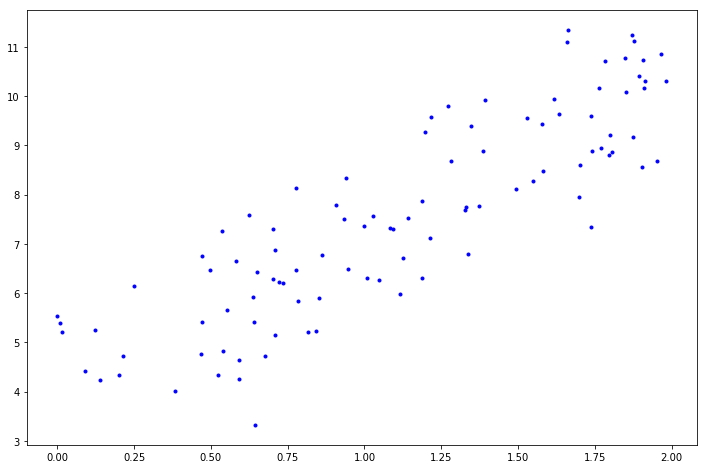array([[4.0939709 ],
[3.08934507]])


array([[ 4.0939709 ],
[10.27266104]])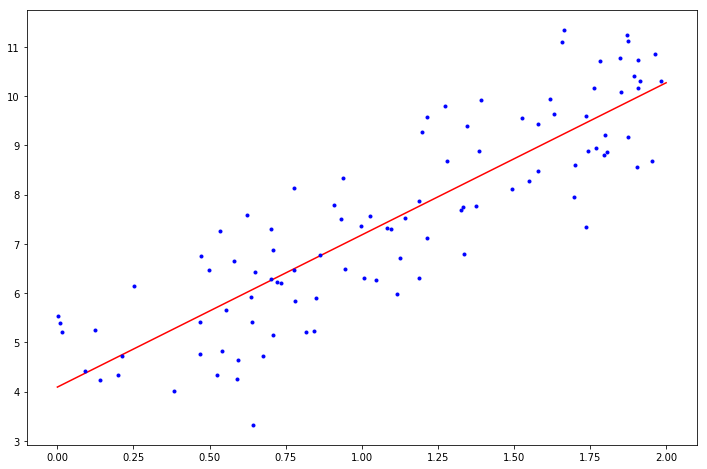LinearRegression(copy_X=True, fit_intercept=True, n_jobs=None,
normalize=False)

(array([4.0939709]), array([[3.08934507]]))

array([[ 4.0939709 ],
[10.27266104]])


### 梯度下降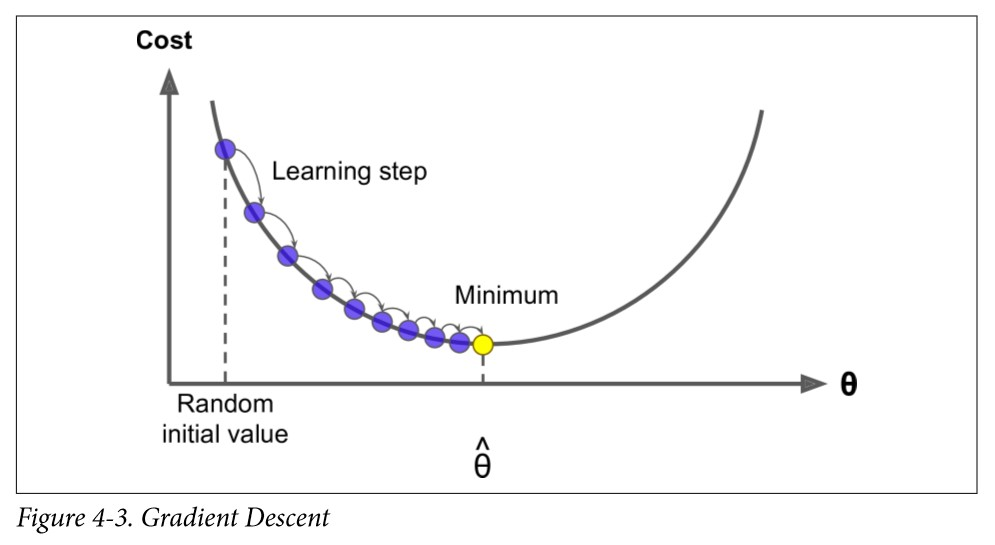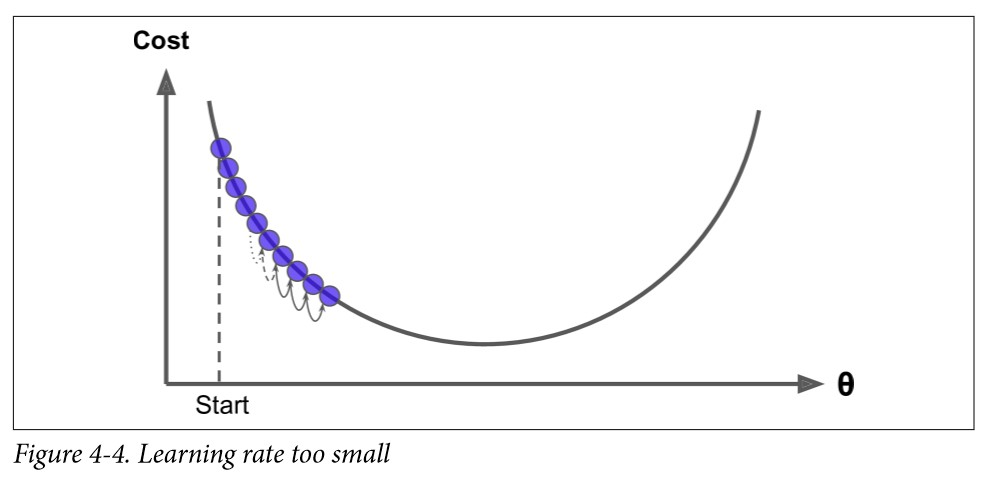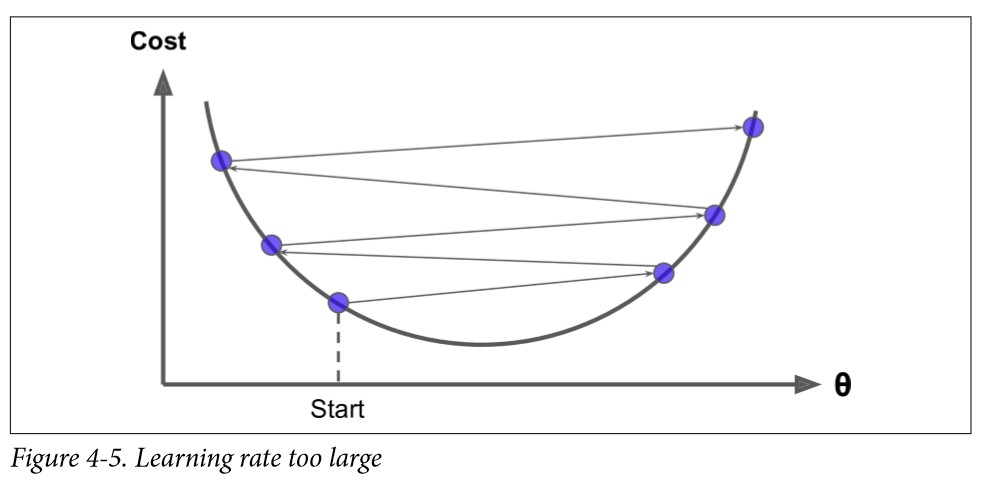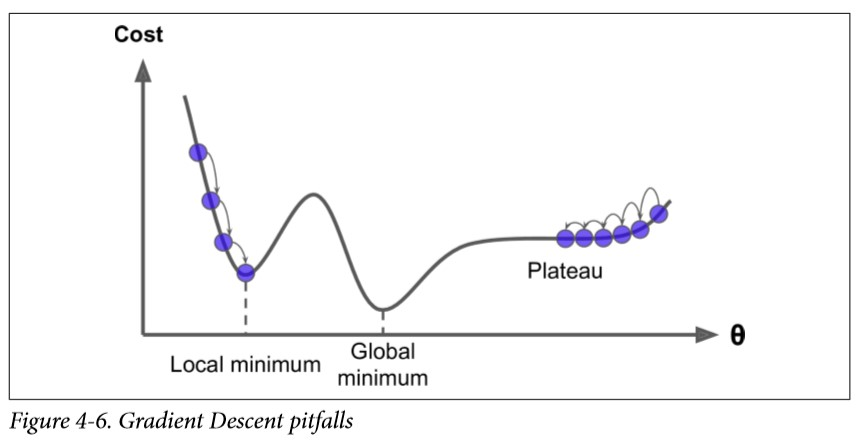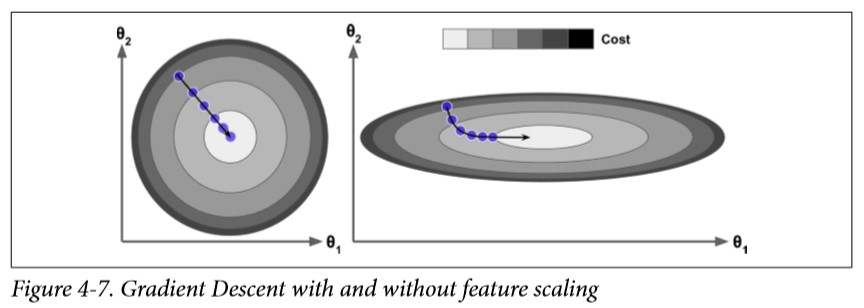### 批量梯度下降

$$\frac{\partial}{\partial \theta_j}MSE(\theta) = \frac{2}{m}\sum_{i=1}^{m} (\theta^T \cdot X^{(i)} - y^{(i)})x_j^{(i)}$$

$$\nabla_\theta MSE(\theta) = \left[ \begin{matrix} \frac{\partial}{\partial \theta_0}MSE(\theta) \\ \frac{\partial}{\partial \theta_1}MSE(\theta) \\ … \\ \frac{\partial}{\partial \theta_n}MSE(\theta) \end{matrix} \right] = \frac{2}{m}X^T \cdot (X \cdot \theta - y)$$

$$\theta^{(next step)} = \theta - \eta\nabla_\theta MSE(\theta)$$

array([[4.0939709 ],
[3.08934507]])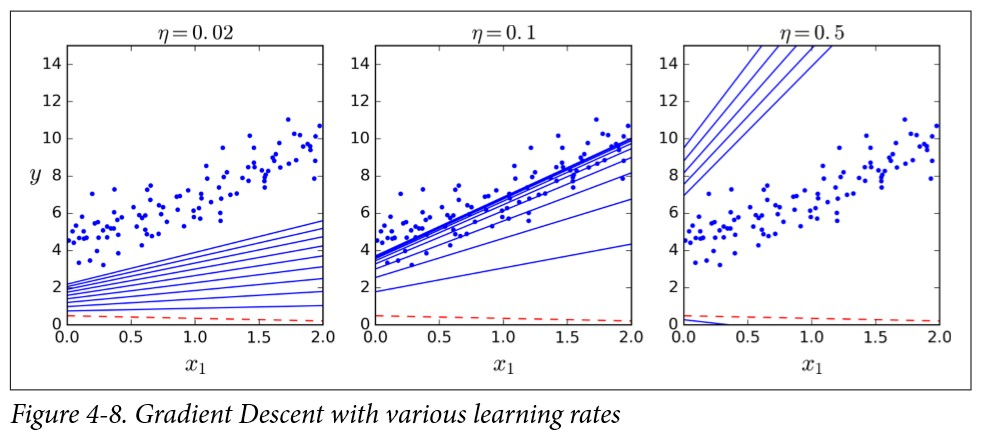### 随机梯度下降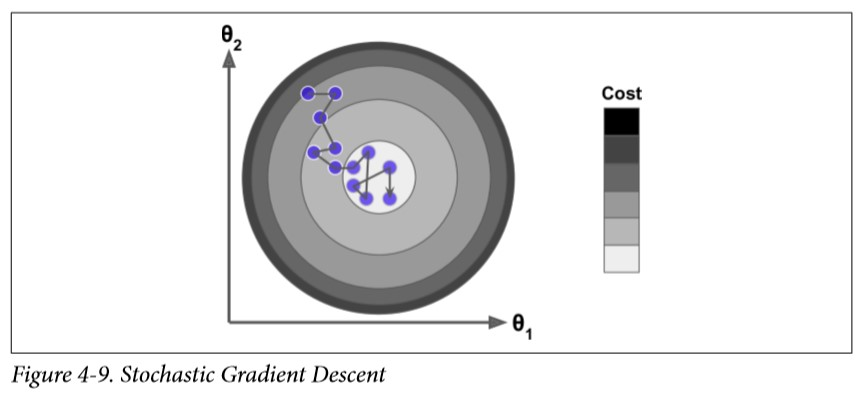array([[4.11135275],
[3.06756448]])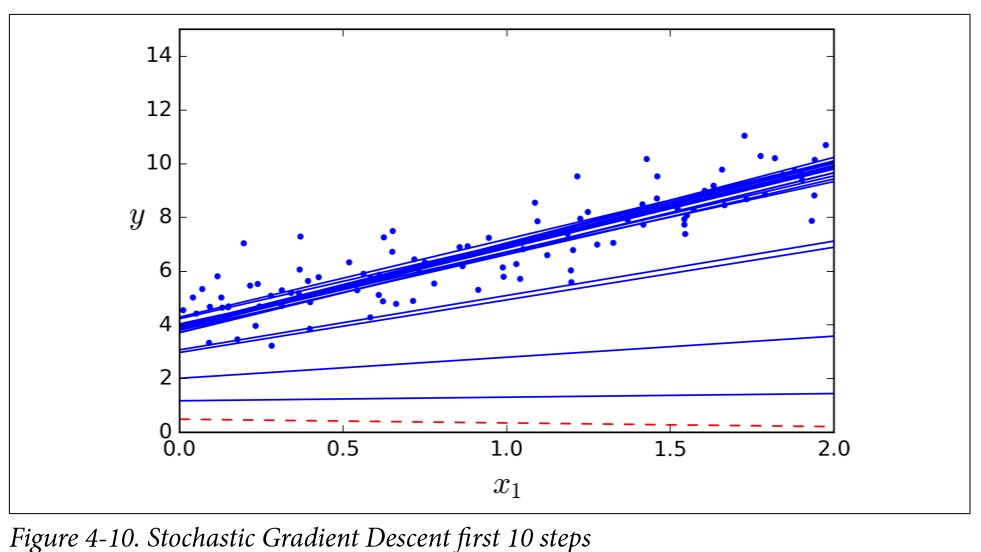Scikit-Learn 里，用 SGD 执行线性回归可以使用 SGDRegressor 类，其默认优化的成本函数是平方误差。下面这段代码从学习率 0.1 开始（eta0=0.1），使用默认的学习计划（跟前面的学习计划不同） 运行了50 轮，而且没有使用任何正则化（penalty=None）：

SGDRegressor(alpha=0.0001, average=False, early_stopping=False, epsilon=0.1,
eta0=0.1, fit_intercept=True, l1_ratio=0.15,
learning_rate='invscaling', loss='squared_loss', max_iter=None,
n_iter=50, n_iter_no_change=5, penalty=None, power_t=0.25,
random_state=None, shuffle=True, tol=None, validation_fraction=0.1,
verbose=0, warm_start=False)


(array([4.08805401]), array([3.08242337]))


### 小批量梯度下降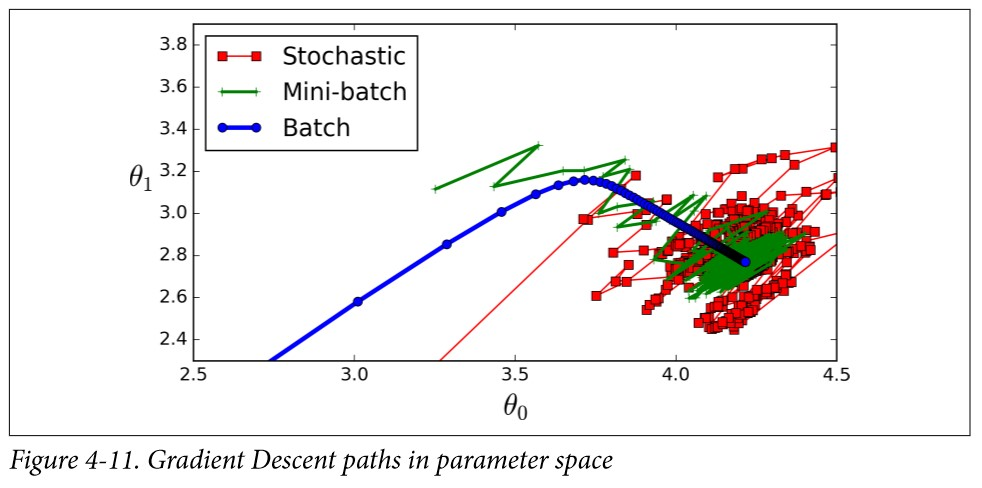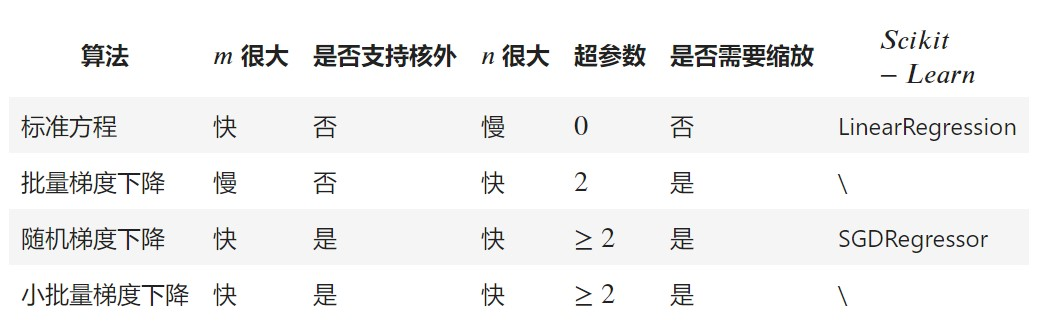### 评论

###### Your browser is out-of-date!

Update your browser to view this website correctly. Update my browser now

×